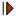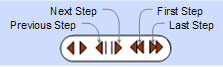# Complete Quadrilateral: Interactive Newton Theorem - Gauss Line. Level: High School, SAT Prep, CollegeProposition. The midpoints M, N, and P of the diagonals AC, BD, and EF of a complete quadrilateral ABCDEF lie on a line called Newton-Gauss Line.

Click the red button () on the figure to start the animation. Drag points A, B, C, D, and F to change the figure. Try the step by step construction.

Complete quadrilateral ABCDEF is the figure determined by four lines, no three of which are concurrent, and their six points of intersection A, B, C, D, E, and F.

Midpoint of a segment: the point on a line segment dividing it into two segments of equal length.

Dynamic Geometry: You can alter the figure dynamically in order to test and prove (or disproved) conjectures and gain mathematical insight that is less readily available with static drawings by hand.

This page uses the TracenPoche dynamic geometry software and requires Adobe Flash player 7 or higher. TracenPoche is a project of Sesamath, an association of French teachers of mathematics.

Instruction to explore the dynamic figure:

• Animation. Click the red buttonto start/stop animation

• Manipulate. Drag points A and C, and line AC to change the figure.

• Step-by-Step construction. Press P and click the left mouse button on any free area to show the step-by-step bar and click 'Next Step' button () to start the construction step-by-step:Hide the step-by-step bar by using again the combination P + click left mouse.Home | Geometry | Collinear Points | Dynamic Geometry | Complete Quadrilateral | Email | By Antonio Gutierrez